How Cheenta works to ensure student success?
Explore the Back-Story

# ISI B.Stat, B.Math Paper 2016 Subjective| Problems & SolutionsHere, you will find all the questions of ISI Entrance Paper 2016 from Indian Statistical Institute's B.Stat Entrance. You will also get the solutions soon of all the previous year problems.

Problem 1:

In a sports tournament of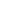players, each pair of players plays exactly one match against each other. There are no draws. Prove that the players can be arranged in an order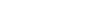such that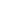defeats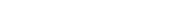Problem 2:

Consider the polynomial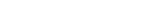where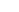is odd and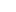is even. Prove that all roots of the polynomial cannot be rational.

Problem 3: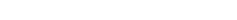is a polynomial with real coefficients.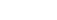Prove that all roots of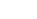cannot be real.

Problem 4:

Let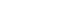be a square. Let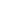lie on the positive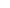-axis and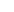on the positive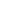-axis. Suppose the vertexlies in the first quadrant and has co-ordinates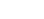Then find the area of the square in terms ofand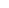.

Problem 5:

Prove that there exists a right angle triangle with rational sides and area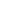if and only if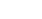andare squares of rational numbers and are in Arithmetic Progression
Hereis an integer.

Problem 6:

Suppose in a triangle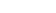,,,are the three angles and,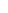,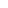are the lengths of the sides opposite to the angles respectively. Then prove that if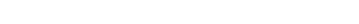then the triangleis isoscelos.

Problem 7: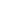is a differentiable function such that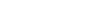where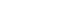.Also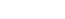.Find the value of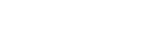Problem 8:

Suppose that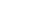is a sequence of real numbers satisfying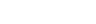.

1. Suppose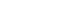, then prove that the sequence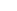is increasing and hence show that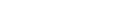.
2. Suppose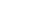, then prove that the sequenceis decreasing and hence show that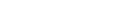.

Here, you will find all the questions of ISI Entrance Paper 2016 from Indian Statistical Institute's B.Stat Entrance. You will also get the solutions soon of all the previous year problems.

Problem 1:

In a sports tournament ofplayers, each pair of players plays exactly one match against each other. There are no draws. Prove that the players can be arranged in an ordersuch thatdefeatsProblem 2:

Consider the polynomialwhereis odd andis even. Prove that all roots of the polynomial cannot be rational.

Problem 3:is a polynomial with real coefficients.Prove that all roots ofcannot be real.

Problem 4:

Letbe a square. Letlie on the positive-axis andon the positive-axis. Suppose the vertexlies in the first quadrant and has co-ordinatesThen find the area of the square in terms ofand.

Problem 5:

Prove that there exists a right angle triangle with rational sides and areaif and only ifandare squares of rational numbers and are in Arithmetic Progression
Hereis an integer.

Problem 6:

Suppose in a triangle,,,are the three angles and,,are the lengths of the sides opposite to the angles respectively. Then prove that ifthen the triangleis isoscelos.

Problem 7:is a differentiable function such thatwhere.Also.Find the value ofProblem 8:

Suppose thatis a sequence of real numbers satisfying.

1. Suppose, then prove that the sequenceis increasing and hence show that.
2. Suppose, then prove that the sequenceis decreasing and hence show that.

This site uses Akismet to reduce spam. Learn how your comment data is processed.

### Knowledge Partner